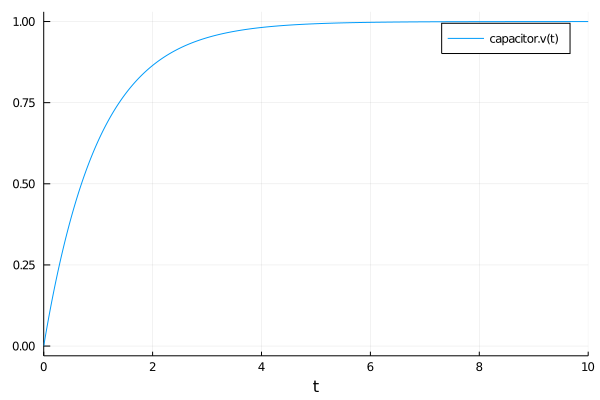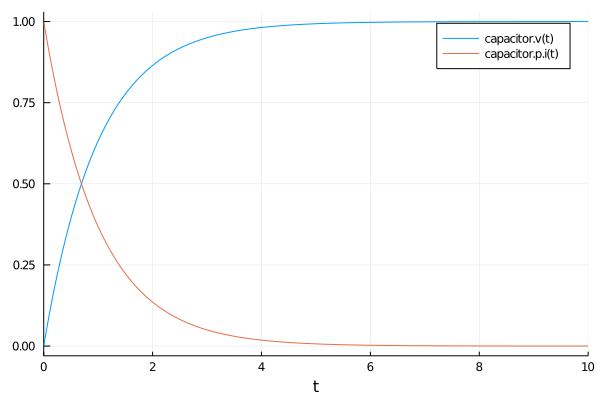# Acausal Component-Based Modeling the RC Circuit

In this tutorial we will build a hierarchical acausal component-based model of the RC circuit. The RC circuit is a simple example where we connect a resistor and a capacitor. Kirchoff's laws are then applied to state equalities between currents and voltages. This specifies a differential-algebraic equation (DAE) system, where the algebraic equations are given by the constraints and equalities between different component variables. We then simplify this to an ODE by eliminating the equalities before solving. Let's see this in action.

## Copy-Paste Example

using ModelingToolkit, Plots, DifferentialEquations

@variables t
@connector function Pin(;name)
sts = @variables v(t)=1.0 i(t)=1.0
ODESystem(Equation[], t, sts, []; name=name)
end

function ModelingToolkit.connect(::Type{Pin}, ps...)
eqs = [
0 ~ sum(p->p.i, ps) # KCL
]
# KVL
for i in 1:length(ps)-1
push!(eqs, ps[i].v ~ ps[i+1].v)
end

return eqs
end

function Ground(;name)
@named g = Pin()
eqs = [g.v ~ 0]
compose(ODESystem(eqs, t, [], []; name=name), g)
end

function OnePort(;name)
@named p = Pin()
@named n = Pin()
sts = @variables v(t)=1.0 i(t)=1.0
eqs = [
v ~ p.v - n.v
0 ~ p.i + n.i
i ~ p.i
]
compose(ODESystem(eqs, t, sts, []; name=name), p, n)
end

function Resistor(;name, R = 1.0)
@named oneport = OnePort()
@unpack v, i = oneport
ps = @parameters R=R
eqs = [
v ~ i * R
]
extend(ODESystem(eqs, t, [], ps; name=name), oneport)
end

function Capacitor(;name, C = 1.0)
@named oneport = OnePort()
@unpack v, i = oneport
ps = @parameters C=C
D = Differential(t)
eqs = [
D(v) ~ i / C
]
extend(ODESystem(eqs, t, [], ps; name=name), oneport)
end

function ConstantVoltage(;name, V = 1.0)
@named oneport = OnePort()
@unpack v = oneport
ps = @parameters V=V
eqs = [
V ~ v
]
extend(ODESystem(eqs, t, [], ps; name=name), oneport)
end

R = 1.0
C = 1.0
V = 1.0
@named resistor = Resistor(R=R)
@named capacitor = Capacitor(C=C)
@named source = ConstantVoltage(V=V)
@named ground = Ground()

rc_eqs = [
connect(source.p, resistor.p)
connect(resistor.n, capacitor.p)
connect(capacitor.n, source.n, ground.g)
]

@named _rc_model = ODESystem(rc_eqs, t)
@named rc_model = compose(_rc_model,
[resistor, capacitor, source, ground])
sys = structural_simplify(rc_model)
u0 = [
capacitor.v => 0.0
]
prob = ODAEProblem(sys, u0, (0, 10.0))
sol = solve(prob, Tsit5())
plot(sol)## Explanation

### Building the Component Library

For each of our components we use a Julia function which emits an ODESystem. At the top we start with defining the fundamental qualities of an electrical circuit component. At every input and output pin a circuit component has two values: the current at the pin and the voltage. Thus we define the Pin component (connector) to simply be the values there:

@connector function Pin(;name)
sts = @variables v(t)=1.0 i(t)=1.0
ODESystem(Equation[], t, sts, []; name=name)
end

Note that this is an incompletely specified ODESystem: it cannot be simulated on its own because the equations for v(t) and i(t) are unknown. Instead this just gives a common syntax for receiving this pair with some default values. Notice that in a component we define the name as a keyword argument: this is because later we will generate different Pin objects with different names to correspond to duplicates of this topology with unique variables. One can then construct a Pin like:

Pin(name=:mypin1)

or equivalently using the @named helper macro:

@named mypin1 = Pin()

Next we build our ground node. A ground node is just a pin that is connected to a constant voltage reservoir, typically taken to be V=0. Thus to define this component, we generate an ODESystem with a Pin subcomponent and specify that the voltage in such a Pin is equal to zero. This gives:

function Ground(;name)
@named g = Pin()
eqs = [g.v ~ 0]
compose(ODESystem(eqs, t, [], []; name=name), g)
end

Next we build a OnePort: an abstraction for all simple electrical component with two pins. The voltage difference between the positive pin and the negative pin is the voltage of the component, the current between two pins must sum to zero, and the current of the component equals to the current of the positive pin.

function OnePort(;name)
@named p = Pin()
@named n = Pin()
sts = @variables v(t)=1.0 i(t)=1.0
eqs = [
v ~ p.v - n.v
0 ~ p.i + n.i
i ~ p.i
]
compose(ODESystem(eqs, t, sts, []; name=name), p, n)
end

Next we build a resistor. A resistor is an object that has two Pins, the positive and the negative pins, and follows Ohm's law: v = i*r. The voltage of the resistor is given as the voltage difference across the two pins while by conservation of charge we know that the current in must equal the current out, which means (no matter the direction of the current flow) the sum of the currents must be zero. This leads to our resistor equations:

function Resistor(;name, R = 1.0)
@named oneport = OnePort()
@unpack v, i = oneport
ps = @parameters R=R
eqs = [
v ~ i * R
]
extend(ODESystem(eqs, t, [], ps; name=name), oneport)
end

Notice that we have created this system with a default parameter R for the resistor's resistance. By doing so, if the resistance of this resistor is not overridden by a higher level default or overridden at ODEProblem construction time, this will be the value of the resistance. Also, note the use of @unpack and extend. For the Resistor, we want to simply inherit OnePort's equations and states and extend them with a new equation. ModelingToolkit makes a new namespaced variable oneport₊v(t) when using the syntax oneport.v, and we can use @unpack avoid the namespacing.

Using our knowledge of circuits we similarly construct the Capacitor:

function Capacitor(;name, C = 1.0)
@named oneport = OnePort()
@unpack v, i = oneport
ps = @parameters C=C
D = Differential(t)
eqs = [
D(v) ~ i / C
]
extend(ODESystem(eqs, t, [], ps; name=name), oneport)
end

Now we want to build a constant voltage electrical source term. We can think of this as similarly being a two pin object, where the object itself is kept at a constant voltage, essentially generating the electrical current. We would then model this as:

function ConstantVoltage(;name, V = 1.0)
@named oneport = OnePort()
@unpack v = oneport
ps = @parameters V=V
eqs = [
V ~ v
]
extend(ODESystem(eqs, t, [], ps; name=name), oneport)
end

### Connecting and Simulating Our Electrical Circuit

Now we are ready to simulate our circuit. Let's build our four components: a resistor, capacitor, source, and ground term. For simplicity we will make all of our parameter values 1. This is done by:

R = 1.0
C = 1.0
V = 1.0
@named resistor = Resistor(R=R)
@named capacitor = Capacitor(C=C)
@named source = ConstantVoltage(V=V)
@named ground = Ground()

Next we have to define how we connect the circuit. Whenever two Pins in a circuit are connected together, the system satisfies Kirchoff's laws, i.e. that currents sum to zero and voltages across the pins are equal. Thus we will build a helper function connect_pins which implements these rules:

function ModelingToolkit.connect(::Type{Pin}, ps...)
eqs = [
0 ~ sum(p->p.i, ps) # KCL
]
# KVL
for i in 1:length(ps)-1
push!(eqs, ps[i].v ~ ps[i+1].v)
end

return eqs
end

Finally we will connect the pieces of our circuit together. Let's connect the positive pin of the resistor to the source, the negative pin of the resistor to the capacitor, and the negative pin of the capacitor to a junction between the source and the ground. This would mean our connection equations are:

rc_eqs = [
connect(source.p, resistor.p)
connect(resistor.n, capacitor.p)
connect(capacitor.n, source.n, ground.g)
]

Finally we build our four component model with these connection rules:

@named _rc_model = ODESystem(rc_eqs, t)
@named rc_model = compose(_rc_model,
[resistor, capacitor, source, ground])

Note that we can also specify the subsystems in a vector. This model is acasual because we have not specified anything about the causality of the model. We have simply specified what is true about each of the variables. This forms a system of differential-algebraic equations (DAEs) which define the evolution of each state of the system. The equations are:

equations(rc_model)

20-element Vector{Equation}:
0 ~ resistor₊p₊i(t) + source₊p₊i(t)
source₊p₊v(t) ~ resistor₊p₊v(t)
0 ~ capacitor₊p₊i(t) + resistor₊n₊i(t)
resistor₊n₊v(t) ~ capacitor₊p₊v(t)
0 ~ capacitor₊n₊i(t) + ground₊g₊i(t) + source₊n₊i(t)
capacitor₊n₊v(t) ~ source₊n₊v(t)
source₊n₊v(t) ~ ground₊g₊v(t)
resistor₊v(t) ~ resistor₊p₊v(t) - resistor₊n₊v(t)
0 ~ resistor₊n₊i(t) + resistor₊p₊i(t)
resistor₊i(t) ~ resistor₊p₊i(t)
resistor₊v(t) ~ resistor₊R*resistor₊i(t)
capacitor₊v(t) ~ capacitor₊p₊v(t) - capacitor₊n₊v(t)
0 ~ capacitor₊n₊i(t) + capacitor₊p₊i(t)
capacitor₊i(t) ~ capacitor₊p₊i(t)
Differential(t)(capacitor₊v(t)) ~ capacitor₊i(t)*(capacitor₊C^-1)
source₊v(t) ~ source₊p₊v(t) - source₊n₊v(t)
0 ~ source₊n₊i(t) + source₊p₊i(t)
source₊i(t) ~ source₊p₊i(t)
source₊V ~ source₊v(t)
ground₊g₊v(t) ~ 0

the states are:

states(rc_model)

20-element Vector{Term{Real, Base.ImmutableDict{DataType, Any}}}:
source₊p₊i(t)
resistor₊p₊i(t)
source₊p₊v(t)
resistor₊p₊v(t)
capacitor₊p₊i(t)
resistor₊n₊i(t)
resistor₊n₊v(t)
capacitor₊p₊v(t)
source₊n₊i(t)
capacitor₊n₊i(t)
ground₊g₊i(t)
capacitor₊n₊v(t)
source₊n₊v(t)
ground₊g₊v(t)
resistor₊v(t)
resistor₊i(t)
capacitor₊v(t)
capacitor₊i(t)
source₊v(t)
source₊i(t)

and the parameters are:

parameters(rc_model)

3-element Vector{Any}:
resistor₊R
capacitor₊C
source₊V

## Simplifying and Solving this System

This system could be solved directly as a DAE using one of the DAE solvers from DifferentialEquations.jl. However, let's take a second to symbolically simplify the system before doing the solve. Although we can use ODE solvers that handles mass matrices to solve the above system directly, we want to run the structural_simplify function first, as it eliminates many unnecessary variables to build the leanest numerical representation of the system. Let's see what it does here:

sys = structural_simplify(rc_model)
equations(sys)

2-element Vector{Equation}:
0 ~ capacitor₊v(t) + resistor₊R*resistor₊i(t) - source₊V
Differential(t)(capacitor₊v(t)) ~ resistor₊i(t)*(capacitor₊C^-1)
states(sys)

2-element Vector{Any}:
capacitor₊v(t)
capacitor₊p₊i(t)

After structural simplification we are left with a system of only two equations with two state variables. One of the equations is a differential equation while the other is an algebraic equation. We can then give the values for the initial conditions of our states and solve the system by converting it to an ODEProblem in mass matrix form and solving it with an ODEProblem mass matrix DAE solver. This is done as follows:

u0 = [
capacitor.v => 0.0
capacitor.p.i => 0.0
]
prob = ODEProblem(sys, u0, (0, 10.0))
sol = solve(prob, Rodas4())
plot(sol)Since we have run structural_simplify, MTK can numerically solve all the unreduced algebraic equations numerically using the ODAEProblem (note the letter A):

u0 = [
capacitor.v => 0.0
]
prob = ODAEProblem(sys, u0, (0, 10.0))
sol = solve(prob, Rodas4())
plot(sol)Notice that this solves the whole system by only solving for one variable!

However, what if we wanted to plot the timeseries of a different variable? Do not worry, that information was not thrown away! Instead, transformations like structural_simplify simply change state variables into observed variables. Let's see what our observed variables are:

observed(sys)

18-element Vector{Equation}:
capacitor₊i(t) ~ resistor₊i(t)
ground₊g₊i(t) ~ 0.0
source₊n₊i(t) ~ resistor₊i(t)
source₊i(t) ~ -resistor₊i(t)
source₊p₊i(t) ~ -resistor₊i(t)
capacitor₊n₊i(t) ~ -resistor₊i(t)
resistor₊n₊v(t) ~ capacitor₊v(t)
resistor₊n₊i(t) ~ -resistor₊i(t)
resistor₊p₊i(t) ~ resistor₊i(t)
capacitor₊p₊i(t) ~ resistor₊i(t)
capacitor₊p₊v(t) ~ capacitor₊v(t)
capacitor₊n₊v(t) ~ 0.0
source₊n₊v(t) ~ 0.0
ground₊g₊v(t) ~ 0.0
source₊v(t) ~ source₊V
source₊p₊v(t) ~ source₊v(t)
resistor₊p₊v(t) ~ source₊v(t)
resistor₊v(t) ~ source₊v(t) - capacitor₊v(t)

These are explicit algebraic equations which can then be used to reconstruct the required variables on the fly. This leads to dramatic computational savings because implicitly solving an ODE scales like O(n^3), so making there be as few states as possible is good!

The solution object can be accessed via its symbols. For example, let's retrieve the voltage of the resistor over time:

sol[resistor.v]

or we can plot the timeseries of the resistor's voltage:

plot(sol, vars=[resistor.v])